AtomicDensityMatrix`
AtomicDensityMatrix`

# OpticalField

OpticalField[ω, a]

gives a complex timedependent electric field with angular frequency ω, real amplitude a, and default polarization.

OpticalField[ω,a,pol]

gives a field with polarization parameters pol.

OpticalField[{ω,k),{a,φ},pol]

gives a spatially dependent planewave field with angular wavenumber k and phase φ.

# Details and Options

• The following options can be given:
•  PolarizationVector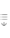{1, 0, 0} reference polarization vector PropagationVector{0, 0, 1} unit wave vector Parameterization"AngleEllipticity" parameterization scheme for polarization CartesianCoordinates{Xx, Yy, Zz} symbols used to represent Cartesian coordinates TimeVariable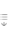Automatic symbol used to represent the time variable
• Possible values for the Parameterization option are "AngleEllipticity", "RatioPhase", and "Stokes".
• The polarization of the field is specified in reference to the unit vectors, given by the value of the option PolarizationVector, and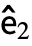=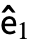, whereis the unit vector specified by the option PropagationVector.
• In the "AngleEllipticity" parameterization, the polarization parameters pol are specified as {α,ϵ}, where α is the polarization angle (relative towith respect to rotation about) and ϵ is the ellipticity. If instead of a list a single value is specified, it is taken as the value of α, with ϵ assumed to be zero.
• In the "RatioPhase" parameterization, the polarization parameters are specified as {χ,ϕ}, where, and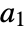and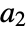are the field amplitudes of the components along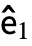and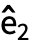, respectively, and ϕ is the relative phase angle between the components. If instead of a list a single value is specified, it is taken as the value of χ, with ϕ assumed to be zero.
• In the "Stokes" parameterization, the polarization parameters are specified as {s1,s2,s3}, where s1, s2, and s3 are the normalized Stokes parameters relative to the axesand.

# Examples

open allclose all

## Basic Examples(1)

Default optical field:

Explicitly specify all parameters in the angle-ellipticity parameterization:

If the ellipticity is omitted, it is assumed to be zero:

## Options(5)

### CartesianCoordinates(1)

Specify variables denoting the Cartesian coordinates:

### Parameterization(1)

Specify polarization using the ratio-phase parameterization:

Specify polarization using the Stokes parameterization:

### PolarizationVector(1)

Specify a reference polarization vector:

### PropagationVector(1)

Specify a unit propagation vector in the direction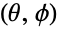. The propagation and polarization vectors should be orthogonal, so we find the polarization vector that is perpendicular to both the propagation vector and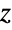:

### TimeVariable(1)

Specify a different time variable: